×
Get Full Access to UNLV - CHEM 121 - Study Guide - Midterm
Get Full Access to UNLV - CHEM 121 - Study Guide - Midterm

×

UNLV / Chemistry / CHEM 121A / What should one study matter?

# What should one study matter? Description

Wednesday, September 21, 2016

## What is matter?CHEMISTRY 121 STUDY GUIDE 1

Chapter One: Matter and Measurement

- Matter — anything that has mass and takes up space (atoms and compounds are  composed of matter)

• Physical properties — can be observed or measured without changing the  composition of the matter

- examples: appearance, color, texture, odor, melting point, freezing point,  solubility, polarity

• Physical changes — changes that do not alter the chemical nature of matter - examples: tearing paper into small pieces, sorting a pile of plastic and metals, ice  melting into water, mixing sand and water, dissolving sugar in water, sublimating  dry ice, melting solid sulfur into liquid sulfur

## What is physical properties?• Chemical properties — changes that alter the chemical nature of matter - examples: reactivity with water, heat of combustion, pH, electromotive force,  flammability, toxicity, oxidation states, chemical stability  Don't forget about the age old question of What is the family of orientation?
We also discuss several other topics like What is resonance in a lewis structure?

• Chemical changes —change that is characterized by the formation of a new  chemical substance

- mixtures —substance created by mixing other substances together defined by the  uniformity of their composition

1

Wednesday, September 21, 2016

• heterogenous — composed of different substances that is made of different  substances that remain physically separate from one another, mixtures of various  proportions of molecules and atoms

## What is physical changes?• homogenous — compounds that make up the mixture are uniform throughout, only  1 of phase of matter observed, atoms/ions are presented in fixed proportions  throughout

- examples: air, sugar water, rain water, vodka, vinegar, dishwashing detergent,  steel

- Math in Chemistry —

• measurement — Terra, Giga, Mega, Kilo, Hecto, Deca, Deci, Centi, Milli, Micro  (symbol = u), Nano, Pico

- Pneumonic Device: The Great Midieval Killer Hates Dragons, Dragons Cry More  Musically Near Pregnancy  If you want to learn more check out What was jj thomson's discovery?

- we are only responsible for knowing Mega-Pico*** If you want to learn more check out What are the 8 aspects that makeup personality?

• significant figures —IT’S ALL ABOUT THE ZEROS!!

- 1. ALL non-zero numbers in a measurement are significant

- 2. ALL middle zeros between non-zero numbers are significant

- 3. Zeros after a decimal point ONLY are significant or a final zero AFTER the  decimal point

- 4. bar notations indicate a significant 0

2

Wednesday, September 21, 2016

• dimensional analysis —used to convert between units of measurement- Quick tip: To ensure that your final answer is in the units requested write the units  on the far right side of the paper where your answer should be. For this problem I  would have written km/hr to indicate the units that my final answer should be in. If you want to learn more check out What controls the type of volcanoes and hazards associated with the volcano?

• density — is equal to mass per unit volume or Density = Mass/Volume - Quick tip: I simply remember the density as DMV… D=M/V.

- Volume = Length * Width * Height (for measured shapes where volume isn’t  provided but needs to be calculating)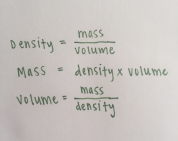3

Wednesday, September 21, 2016

Chapter Two: Atoms, Molecules and Ions

- Laws that led to the Atomic view of Matter

• Law of Conservation of Mass — total mass of the reactants will equal the mass of  the products in every chemical reaction

• Law of Definite (or Constant) Composition — the number of atoms and relative  numbers are constant in a given compound

• Law of Multiple Proportions — if 2 elements combine together to form 1 compound,  A+B will be equivalent to small WHOLE numbers

- History of Atoms  Don't forget about the age old question of Why are lines parallel when they have the same slope?

- Dalton’s Atomic Theory — John Dalton introduced the idea that all matter is made up  of atoms

• Dalton expressed that atoms of 1 element have the same mass and property make up than atoms of a different element

• Dalton believed atoms are the fundamental building blocks of nature that could not  be split apart into smaller particles (we now know that atoms can be split into  smaller particles such as electrons, neutrons, protons, cathode rays and  radioactivity)

• in chemical reactions, atoms would rearrange themselves and combine with other  atoms in new ways

4

Wednesday, September 21, 2016

• J.J.Thomson’s plum pudding model (“Raisin Muffin” Model) — a positive sphere  (pudding) of matter with negative electrons (plums) spread in layers throughout the  sphere

• Thompson measured ratio of cathode ray particles mass to its charge using  magnetic and electric fields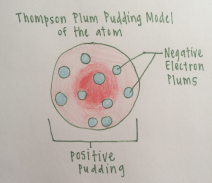- Ernest Rutherford’s a- scattering experiment gained him the discovery of the atomic  nucleus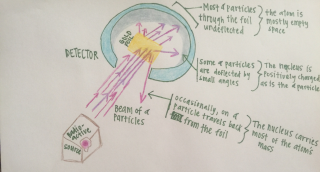-

5

Wednesday, September 21, 2016

- Atomic Symbols and Atomic Mass — Found using the periodic table of elements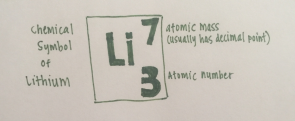- The Atomic Mass often translated into the measurement grams represents the  average mass of the atomic element (7 g Li)

- The Atomic Number represents the number of (3) protons and (3) electrons in an  elements atom (Li)

- Neutrons are calculated by taking the atomic number and subtracted it by the atomic  mass (Lithium: 7 - 3 = 4 neutrons)

- Quick Tip: It is highly recommended that the most used elements’ names and  symbols are memorized. Even better if you can name them all! This is important to  finding the correct mass number on a periodic table that does not have the full name  of the element on it. The one provided for our exam will not have element’s names  printed on it

- Periodic Table — Discovered by Mendeleev the father of the periodic table of  elements

• Periodic Law of the Elements — elements of similar properties occur at periodic  intervals when arranged in a particular order

6

Wednesday, September 21, 2016

- Groups — vertical columns

• 1 A = Alkali Metals

• 2 A = Alkaline Earth Metals

• 6 A = Chalcogens

• 7 A = Halogens

• 8 A = Noble gases

- Periods — horizontal rows

- metalloids —Boron, Silicon, Geranium, As, Sb and Te

- nonmetals —Hydrogen, Group 8A, Group 7 A, Oxygen, Sulfur, Carbon, Nitrogen,  and Phosphorus

- metals — transitional metals are located predominantly in the middle Group 3 B  through 2B with Group 1 A (excluding Hydrogen), Group 2 A, Group 3 A (not  including Boron), Sn, Pb, Bi and Po making up the main-group metals

- Ionic Compounds — bonds formed through charge transfer (unequal electron  sharing)

- forms an ion primarily between a metal and a nonmetal

• predicting formulas —to predict a compounds formula it is imperative that one  knows the charge of each of the most popular elements used in compounds

7

Wednesday, September 21, 2016

• neutral species of compounds have electrons lost and gained at equal values  (negative and positive ions will cancel each other out creating neutrality)

• Cation (+) is named first followed by the anion (-)

• charges are not shown in the compound name

• subscripts in the formula represent the combining ratio for the ions - Cations are positively charged ions

• Common cations to know: H+, Li+, Na+, K+, Cs+, Ag+, NH4 +, Cu +, Co 2+,  Cu 2+, Fe 2 +, Mn 2+, Hg2 2+, Hg 2+, Ni 2+, Pb 2+, Sn 2+, Mg 2+, Ca 2+,Sr  2+, Ba 2+, Zn 2+, Al 3+, Cr 3 +, Fe 3+

- Quick tip: The bolded cations are the most used throughout this course - Anions are negatively charged ions

• Common Anions to know: H-, F-, Cl-, Br-, I-, CN-, OH-, CH3COO-, ClO3-,  ClO4 -, NO3-, MnO4-, O 2-, O2 2-, S2-, CO3 2-, CrO4 2-, Cr2O7 2-, SO4 2-, N  3-, PO4 3-

- Quick Tip — the bolded terms represent the most used anions throughout  this course

• Trends on the periodic table can be used to determine ionic charges - Group 1A has a 1+ or just + charge, 2 A has a 2+ charge, N has a 3-, most of  Group 6 A has a 2- charge, most of Group 7 A has a - charge, Al has a 3+  charge

8

Wednesday, September 21, 2016

- Group 8 A consists of noble gases which are stable on their own and  therefor have a neutral charge

• nomenclature — for two binary elements (metals with fixed charge)- First word — full name of metal

- Second word — base name or stem word in element followed by “-ide” • Ex: (Mg(OH)2) = Magnesium Hydroxide

• nomenclature — metals with unfixed charge

- Frist word — full name of metal followed by the roman numeral of the charge on  the metal

- Second word — stem word of anion followed by “-ide”

• Ex: iron (III) sulfide (Fe2S3)

• polyatomic ions — groups of atoms held together by covalent bonds • MUST MEMORIZE PER DR. VO!!!

9

Wednesday, September 21, 2016

- NH4 + Ammonium, H3O + Hydronium, CH3COO- Acetate, CN - Cyanide, OH Hydroxide, ClO3- Chlorate, ClO4- Perchlorate, NO2 - NItrite, NO3 - Nitrate,  MnO4- Permanganate, CO3 2- Carbonate, Cr2O7 2- dichromate, SO4 2- Sulfate,  SO3 2- Sulfite, PO4 3- Phosphate

- Quick tip: I recommend making flash cards for the polyatomic ions to ensure that  the name, formula and charge is memorized because they will be used later in  stoichiometry problems, balancing equations, etc….

- Covalent compounds — bonds formed through sharing electrons  • primarily involving nonmetals

• nomenclature

- First word — Greek prefix + name of 1st element

• prefix “mono” is not included

• when the element name begins with a vowel, the A or O at the end of Greek  prefix is dropped for pronunciation reasons

- Second Word — Greek prefix + base/stem name of 2nd element ending in “ide” • Ex: CO2 — Carbon dioxide

• When hydrogen is first in a compound, it takes the place as a metal and does  not need the greek prefix added to its name

- Ex: Hydrogen phosphate (H3PO4)

10

Wednesday, September 21, 2016

- Acids to memorize

• HCl (aq) hydrochloric acid, HNO3(aq) nitric acid, H2SO4 (aq) sulfuric acid,  H3PO4 (aq) phosphoric acid

- (properties above are all acids made up of compounds dissolved in  water)

Chapter 3: Stoichiometry

- The Mole —1 mole of anything is equal to Avogadro’s Number = 6.022 * 10^23 of  anything

• this number makes working with large numbers easier

• Ex: 12 g C is equal to 1 mol which is equal to 6.022 * 10^23 particles of C - Empirical Formulas —formula giving the simplest positive whole integer ratio of  atoms in a compound

• 1. — take each of the given masses and convert to moles

• 2. — Divide each element’s mol by the lowest mol value of the elements

• 3. — If each number is a whole number or very close then these numbers  represent the ratio formula for each element within the compound

• 4. — If one or more numbers aren’t whole numbers, multiply each by a number that  will bring the decimals to whole numbers (as in the example below)

11

Wednesday, September 21, 2016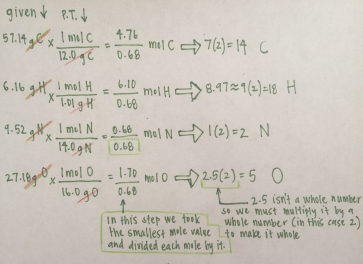• 5. — Write out the formula C14H18N2O5

- Molecular Formulas (combustion) —

- molecular formulas- the formula for a compound

• ex: A compound is 75.46% carbon, 20.10% ocygen, and 4.43% hydrogen by  mass. It's molecular weight is 318.31 g/mol.

- 1. Find empirical formula

• Find mass of each element by fixing each overall mass sample with 100  g (you can use any mass but 100 works best with percentages)  • (.7546) (100 g) = 75.46 g C

• (.0443) (100 g) = 4.43 g H

• (.2010) (100 g) = 20.10 g O

• Convey mass of each element to moles

12

Wednesday, September 21, 2016

• (75.46 g C) (1 mol/ 12.00 g C) = 6.289 mol C

• (4.43 g H) (1 mol/ 1.008 g H) = 4.39 mol H

• (20.10 g O) (1 mol/ 16.00 g O) = 1.256 mol O

• Ratio of moles of each element

• (1.256 mol O)/ (1.256) = 1 mol O

• (6.289 mol C)/ (1.256) = 5.007 mol C

• (4.39 mol H)/ (1.256) = 3.50 mol H

• Multiply each value by 2 to achieve whole numbers for each value  • The empirical formula is C10H7O2

- 2. Calculate the mass of the empirical formula

• 10(12.00) + 7(1.008) + 2(16.00) = 159.06 g/mol

- 3. Determine how many empirical units are in a molecular unit by dividing the  molecular mass by the empirical one

• (318.31 g/mol)/(159.06 g/mol) = 2.001 empirical units per molecular  - 4. New formula for molecular unit

• C20H14O4

- Chemical Equations —

• writing from scratch —writing chemical formula from scratch

- when writing chemical equations it is important to identify the charge of each  element within a compound as well as which compound or element is the cation  (+) and which is the anion (-)

13

Wednesday, September 21, 2016

• Ex: sodium hydroxide and iron (II) chloride react with each other to form  sodium chloride and iron (II) hydroxide

- 1. Write out each compound and add subscripts to balance the charges  NaOH (aq) + FeCl2 (aq) ----> NaCl(aq) + Fe(OH)2 (s) • he products were formed simply by taking the cations, rewriting  them in the same order and then switching the anions. A cation  will always be with an anion within a compound

- 2. Now simply balance the equation so that each side has an equal  amount of elements on both sides

2NaOH(aq) + FeCl2 ----> 2NaCl (aq) + Fe(OH)2 (s) - balancing — As seen above, placing numbers in front of entire compound so  that each element has the same amount in both the products and the reactants  • Quick tip — Subscripts within a chemical equation should NEVER be  changed

• Stoichiometry —

- 1. Write the correct balanced chemical equations with the correct formulas - 2. Identify the given and unknown quantities for each

• Units given must be converted to moles

- 3. Use coefficients within the chemical equation to set up mole ratios - 4. After solving, convert quantities into the required units (typically grams)

14

Wednesday, September 21, 2016

- 5. Make sure that the answer is reasonable and the units are correct - basic —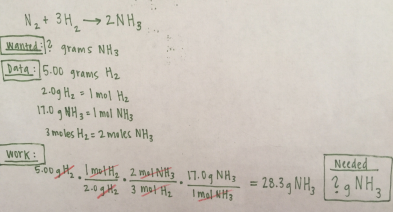- limiting reactant — Perform multiple stoichiometry problems with the different  amounts of grams given to determine which elements limits the overall product  yield.

15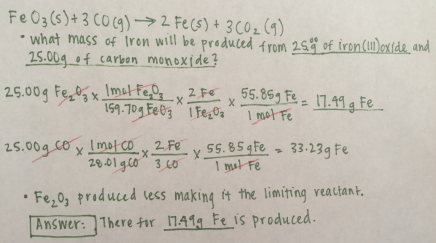Wednesday, September 21, 2016

• Quick tip: In this limiting reactant problem, we were given only 2 values to work  with (FeO3 and CO). Some problems may involve 3 or more values. The  process would still be the same with the smallest value representing the  limiting reactant

- percent yield —Usually the total yield is calculated in grams using a stoichiometry  problem method and then divided by a theoretical yield that will be given within a  problem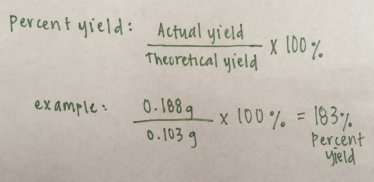16

Page ExpiredIt looks like your free minutes have expired! Lucky for you we have all the content you need, just sign up here